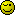# Else statement did not work

Hi

I encountered a problem in my if,elseif and else statement.

I have this code:

``````
\$HDMF = \$_POST['HDMF'];

if(\$TotEarn <= 1500){
\$HMDF = round((\$TotEarn * 0.01), 2);
}
elseif (\$TotEarn >= 1501) {
\$HDMF = round((\$TotEarn * 0.02), 2);
}
else {
\$HDMF = (0);
}
\$smarty->assign('HDMF', \$HDMF);

``````

I have \$TotEarn = 893.5 so that my \$HDMF will be 0 right?

But it become blank or empty.

What’s wrong in my condition.

Thank you

Are you sure? Did you echo \$TotEarn?

Why?
If \$TotEarn = 893.5 , what part of your if-elseif-else should be executed? Put some echoes in there to watch the flow.

What becomes blank? You don’t see anything on the screen? Maybe you’re telling Smarty to do zero surpression (a zero values is displayed as blank) ? I don’t know Smarty, so I’m just guessing here.
Do an echo of \$HDMF after the if-elseif-else to check its value.

if \$TotEarn = 893.5 it means that it less than 1500, so first condition should work.
and if \$TotEarn defined in the code somehow?

i tried to var_dump the \$TotEarn and \$HDMF and the result is \$TotEarn = 893.5 and \$HDMF = Null

I want to execute is the else condition because it is <= 1500 and also it is not >= 1500 right?

So it should be zero…

I tried this code:

``````
\$HDMF = \$_POST['HDMF'];

if(\$TotEarn >= 100 && \$TotEarn <= 1500){
\$HMDF = round((\$TotEarn * 0.01), 2);
}
elseif (\$TotEarn >= 1501) {
\$HDMF = round((\$TotEarn * 0.02), 2);
}
else {
\$HDMF = (0);
}

``````

if I used this code the 893.5 will be used the if condition right???
but now the output is an empty data

Thank you

The computation is like this:

if \$TotEarn is 1500 and below the share is 1% of \$TotEarn
and if \$TotEarn is above 1500 the share is 2% of \$TotEarn

Whats wrong in my code why does the below 1500 did not get the 1% of the \$TotEarn but the output is empty data
Thank you

Maybe because you keep spelling \$HDMF differently?

If this is the case, then the lesson is to name your variables with a bit more thought.

If this is the computation, then why don’t you code it like that?

``````
if (\$TotEarn <= 1500) {
\$HDMF = round((\$TotEarn * 0.01), 2);
} else {
\$HDMF = round((\$TotEarn * 0.02), 2);
}

``````

And like Cups says, pay attention to “little” details like variable names.

Yes, Thank you…
I change my code from this:

``````
\$HDMF = \$_POST['HDMF'];

if(\$TotEarn <= 1500){
\$HDMF = round((\$TotEarn * 0.01), 2);
}
elseif (\$TotEarn > 1500) {
\$HDMF = round((\$TotEarn * 0.02), 2);
}
else {
\$HDMF = (0);
}

``````

is it right for if \$TotEarn = 1500 and below

and \$TotEarn = above 1500

Thank you

Okay i try your code, it also work thank you

Thank you

Yeah, didn’t mean to sound so preachy there, just saying its fine to make errors like that as long as you address the underlying cause – and sometimes there is a bonus to naming variables longhand – yes, they seem to take up more space but reading

\$highDensityMoneyFactor = 0;

might be more:

Then again you might simplify it to \$bonus.If you find that way of thinking to be in any way at all cool or helpful then you might like to get hold of a copy of “Clean Code” by Uncle Bob Martin as your xmas treat (nods to Anthony Sterling).